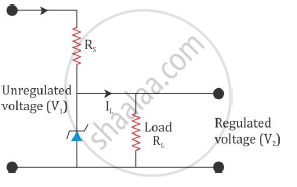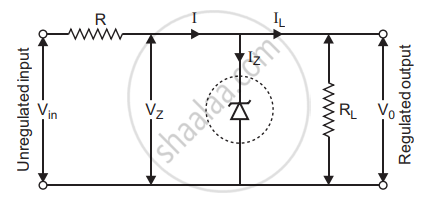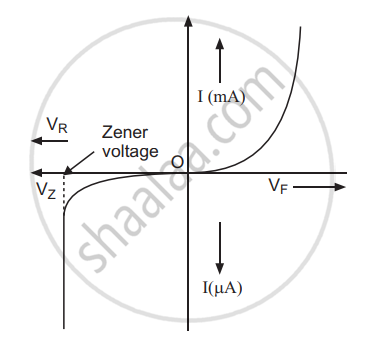# Describe, with the Help of a Circuit Diagram, the Working of Zener Diode as a Voltage Regulator. - Physics

Describe, with the help of a circuit diagram, the working of Zener diode as a voltage regulator.

How Zener diode used as a voltage regulator?

#### Solution 1

Zener diode as a voltage regulator:

To get a constant d.c. the voltage from the d.c. the unregulated output of a rectifier, we use a Zener diode. The circuit diagram of a voltage regulator using a Zener diode is shown in the figure below.The unregulated d.c. voltage is connected to the Zener diode through a series resistance Rs such that the Zener diode is reverse biased. If the input voltage increases, the current through Rs and the Zener diode also increases. This increases the voltage drop across Rs without any change in the voltage across the Zener diode. This is because the Zener voltage remains constant in the breakdown region even though the current through it changes.

Similarly, if the input voltage decreases, the current through Rs and the Zener diode also decreases. The voltage drop across Rs decreases without any change in the voltage across the Zener diode. Thus, any increase or decrease in the input voltage results in an increase or decrease of the voltage drop across Rs without any change in the voltage across the Zener diode. Hence, the Zener diode acts as a voltage regulator.

#### Solution 2

The Zener diode makes its use as a voltage regulator due to the following property :

When a Zener diode is operated in the breakdown region, the voltage across it remains practically constant for a large change in the current.

A simple circuit of a voltage regulator using a Zener diode is shown in the figure. The Zener diode is connected across load such that it is reverse biased.The series resistance R absorbs the output voltage fluctuations so as to maintain constant voltage across the load.

If the input dc voltage increases, the current through R and Zener diode also increases. So, voltage drop across R increases, without any change in the voltage across zener diode.

I-V CharacteristicsConcept: Zener Diode as a Voltage Regulator
Is there an error in this question or solution?